# Evaluation AC3-12

## Comparison of Test Data and CFD

A rather good agreement between the experiments and predictions was obtained for gas and particle phase in both swirling cases considered. The comparison of the calculated streamlines of the gas flow with those obtained from the integration of the measured axial velocity shows that the flow field is predicted reasonably well for both conditions (Figure 10). The most obvious difference is that the axial extension of the central recirculation bubble is predicted to be larger at the top and downstream ends for both cases. The predicted width of the central recirculation bubble and the extension of the recirculation at the edge of the pipe expansion are in good agreement with the measured results.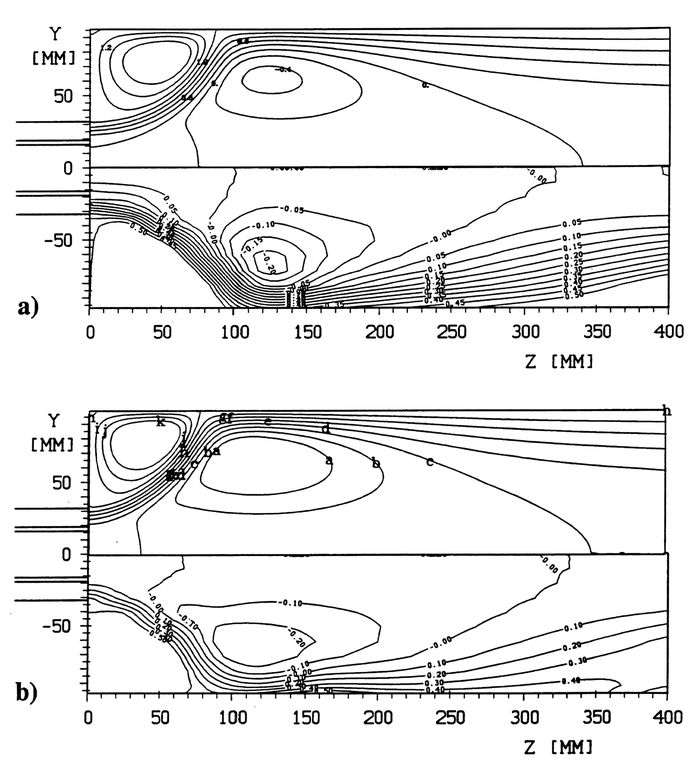Figure 10: Measured and calculated gas-phase streamlines (the upper parts of each figure corresponds to the calculations and the lower parts show the measurements); (a) Case 1; (b) Case 2.

The measured cross-sectional profiles of the three velocity components are compared with the calculations in Figure 11 for Case 2. The agreement is very good, except for the tangential velocity which is under-predicted in the region downstream of the location where the recirculation bubble has its largest radial extension. Although, the turbulent kinetic energy of the gas phase is considerably under- predicted in the initial mixing region between the primary and annular jets and within the recirculation at the edge of the pipe expansion (z = 52 mm), the agreement is reasonably good for the cross-sections further downstream. Similar results have been obtained for swirl Case 1 which was summarized in a previous publication (Sommerfeld et al. 1992).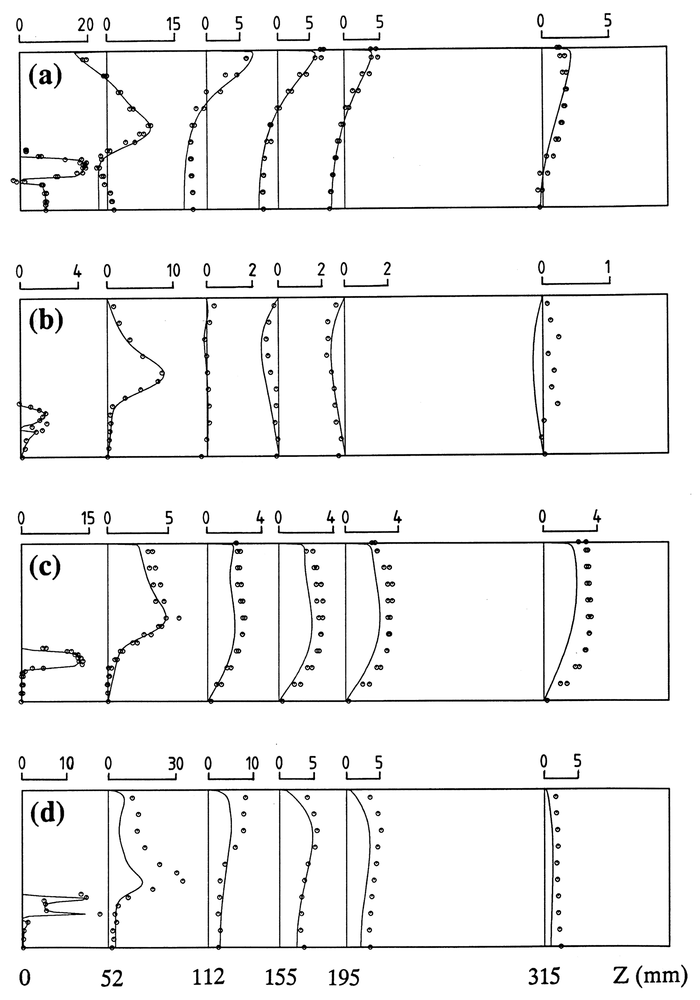Figure 11: Comparison between measurements and numerical calculations for the gas-phase in Case 2: (a) axial mean velocity; (b) radial mean velocity; (c) tangential mean velocity; (d) turbulent kinetic energy.

The measured and calculated particle mean velocities and the associated velocity fluctuations are compared in Figures 12 and 13 again for case 2. All mean velocity components are generally well-predicted, except for the radial velocity which is predicted to be positive at z = 315 mm (i.e. the particles move towards the wall) whereas the experiments show negative velocities. This implies that in the experiment the particles move on average away from the wall. Although, it might be expected that the numerical results in the near-wall region are very sensitive with respect to the modelling of the wall collision process, it was found that a variation in the normal restitution ratio and the friction coefficient in the three-dimensional inelastic collision model did not result in considerable changes in the velocity profiles. The scattering of the numerical results in the core region downstream of the location, where the central recirculation bubble has its largest radial extension is a result of the low number of collected particles, since the majority of the particles have already moved out of the core region.

The particle velocity fluctuations are more or less under-predicted for all three velocity components, which presumably is caused by the under-prediction of the gas-phase turbulent kinetic energy in the initial region of flow development (Figure 11(d)). This is demonstrated in Figure 12(b) for the fluctuation of the axial particle velocity component.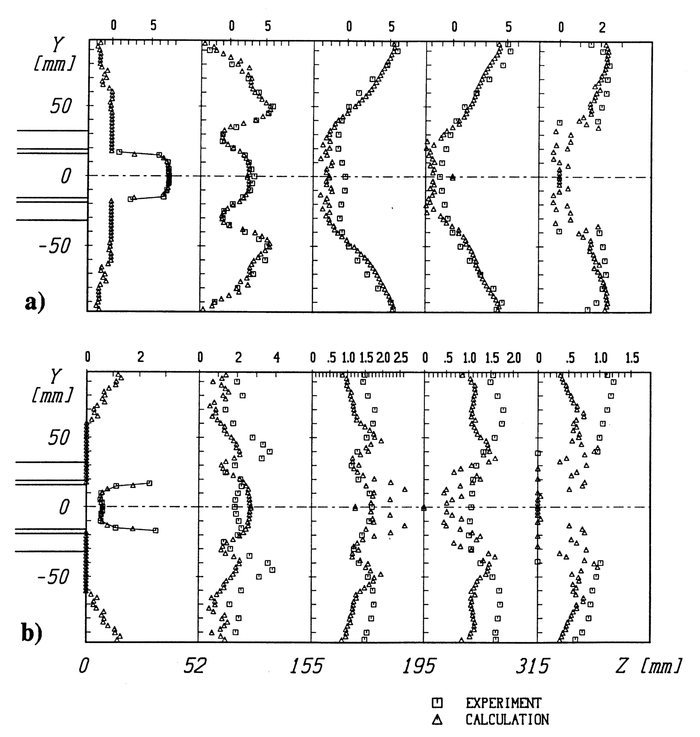Figure 12: Comparison of measured and calculated axial particle velocity profiles: (a) mean particle velocity (m/s); (b) mean particle velocity fluctuation (m/s), Case 2.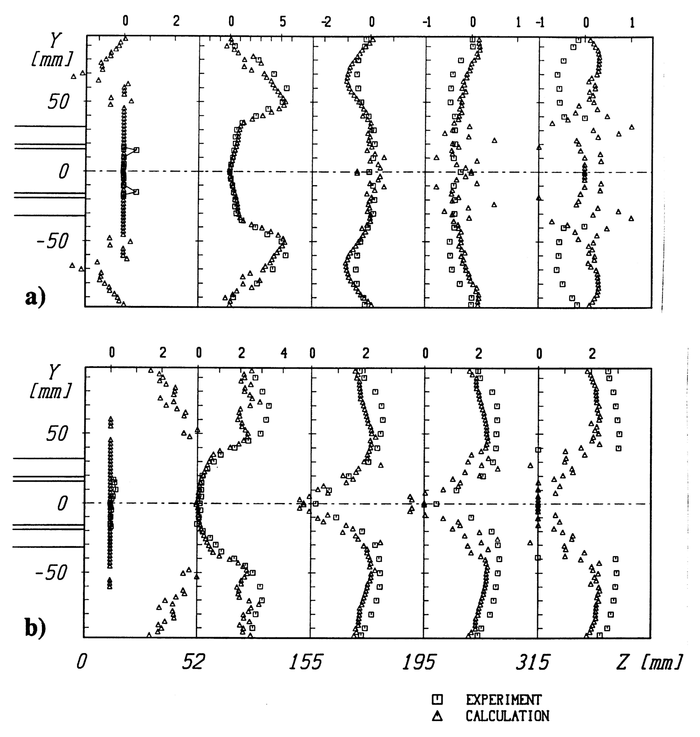Figure 13: Comparison of measurements and calculations: (a) radial particle velocity (m/s); (b) tangential particle velocity (m/s), Case 2.

The calculated particle mass flux shows very good agreement with the experiments in the initial region just downstream of the inlet, namely at z = 52 mm, and further downstream at z = 315 (Figure 14(a)). In the intermediate region, where the particles rebound from the wall and interact again with the central recirculation bubble (see Figure 7), some differences between the experiments and predictions are observed in the near-wall region. At z = 155 and 195 mm, the measurements show a relatively narrow layer of particles near the wall with the maximum being directly at the wall. The calculations show a slightly wider particle layer with a maximum in the particle mass flux located at a radial position of 65 mm, which might result from the assumptions made in the particle-wall collision model.

A sensitivity study, by using different normal restitution ratios and wall friction coefficients in the particle-wall collision model, shows that their influence on the particle mass flux distribution at z = 155 and 195 mm is not too strong (Figure 15). A reduction in the normal restitution ratio from 0.8 to 0.6 gives a slightly narrower particle layer with a more pronounced maximum closer to the wall. When the particle lift force is switched off in the calculations, the particle layer becomes wider with a less pronounced maximum in the mass flux distribution near the wall. This also shows that the particle lift force due to rotation is only important in the near-wall region, where high rotational velocities are induced by the wall collision. Since the particles lag behind the air flow in the near-wall region, the direction of the lift force is directed towards the centreline. From these results, one may conclude that the particle motion in the near- wall region might also be affected by electrostatic forces, although the Plexiglas test section was carefully grounded.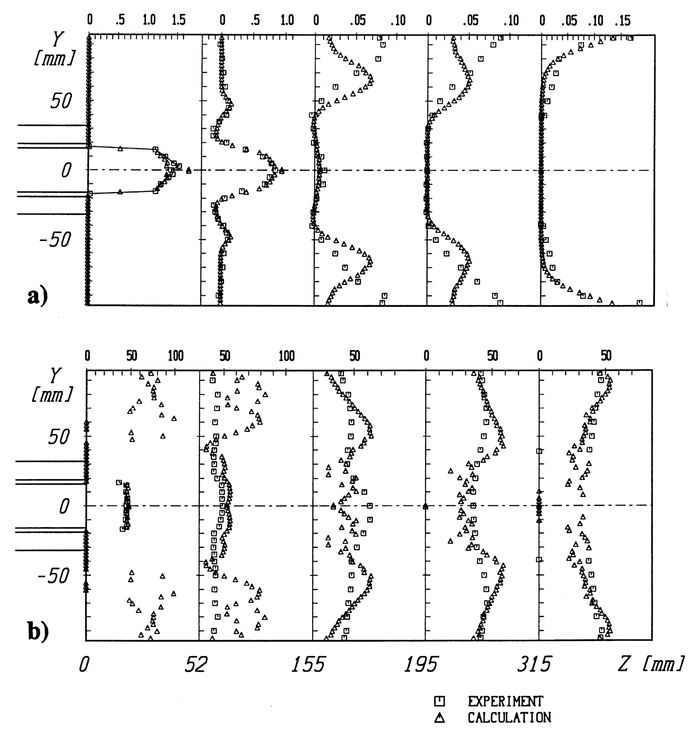Figure 14: Comparison of measurements and calculations: (a) stream-wise particle mass flux (kg/m2s); (b) particle number mean diameter (μm), Case 2.

In the publications of Sommerfeld et al. (1992) additional numerical results for the swirl Case 1 may be found. Details on measured and calculated particle size-velocity correlations are presented in Sommerfeld and Qiu (1993).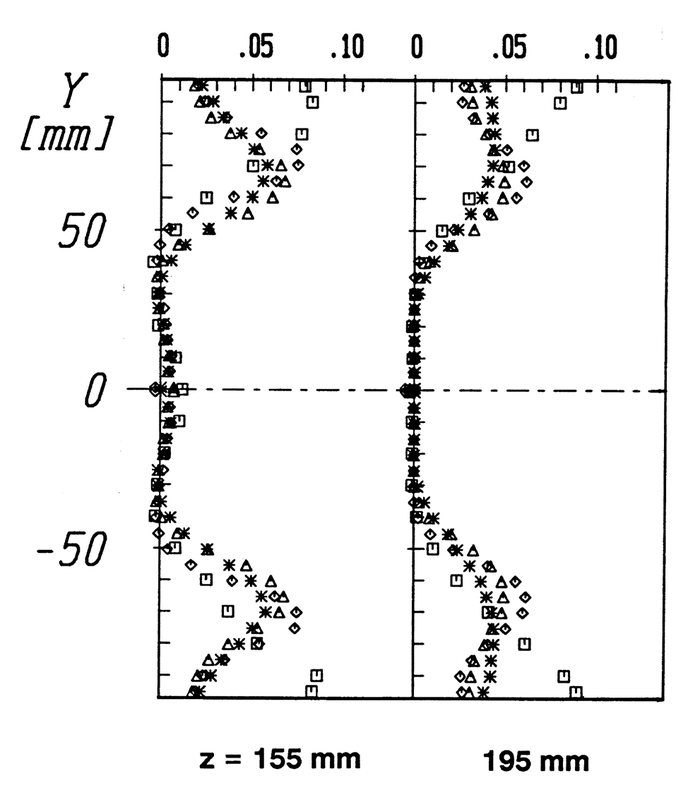Figure 15: Measured and calculated axial particle mass flux at z = 115 and 195 mm for different parameters in the wall collision model: △, with lift force, e = 0.8, μ = 0.3; ◊, with lift force, e = 0.6, μ = 0.3; ✱, without lift force, e = 0.8, μ = 0.3; □, experiment, Case 2.

The test case particle-laden swirling flow (here Case 1) was also used for validation of "in-house" codes at the 5th Workshop on Two Phase Flow Predictions (Sommerfeld and Wennerberg 1991). It should be noted that in all the graphs shown below, the swirling Case 1 described above is named Case 3. Several groups have participated in these calculations and the results may be found in the Workshop Proceedings, including a description of the numerical methods applied.

Most of the calculations were performed with the two-dimensional Euler/Lagrange approach (e.g. Azevedo/Pereira, Milojevic, Wennerberg, Berlemont, Blümcke and Ando/Sommerfeld). Regarding the fluid flow mostly the standard k-ε turbulence model was used, some participants adapted however the model constants (i.e. Blümcke). Moreover, some contributions were based on the application of algebraic stress models in order to predict the stress components of the fluid, which were also used for the particle tracking (e.g. Berlemont and Wennerberg). The two-fluid approach was only applied by Simonin also using a k-ε turbulence model. The fluctuating motion of the dispersed phase was linked to the continuous phase turbulence through analytic correlations (Simonin 1991).

Some results of the test case calculations for Case 1 (in the figures named Case 3) are shown below (Fig. 16 to 23), revealing that there is a noticeable scatter of the calculations performed by the different groups. The mean velocities for gas and particle phase are captured reasonably well, but all components of the fluctuating velocities are generally considerably under-predicted, for both gas and particles. In the numerical calculation of the particle mass flux profiles the critical issue is the prediction of the correct penetration of the particles into the central recirculation region. At the end of the recirculation region mostly the particle mass flux is under-predicted. Surprisingly, the profiles of the particle number mean diameter are captured quite well by most of the computations.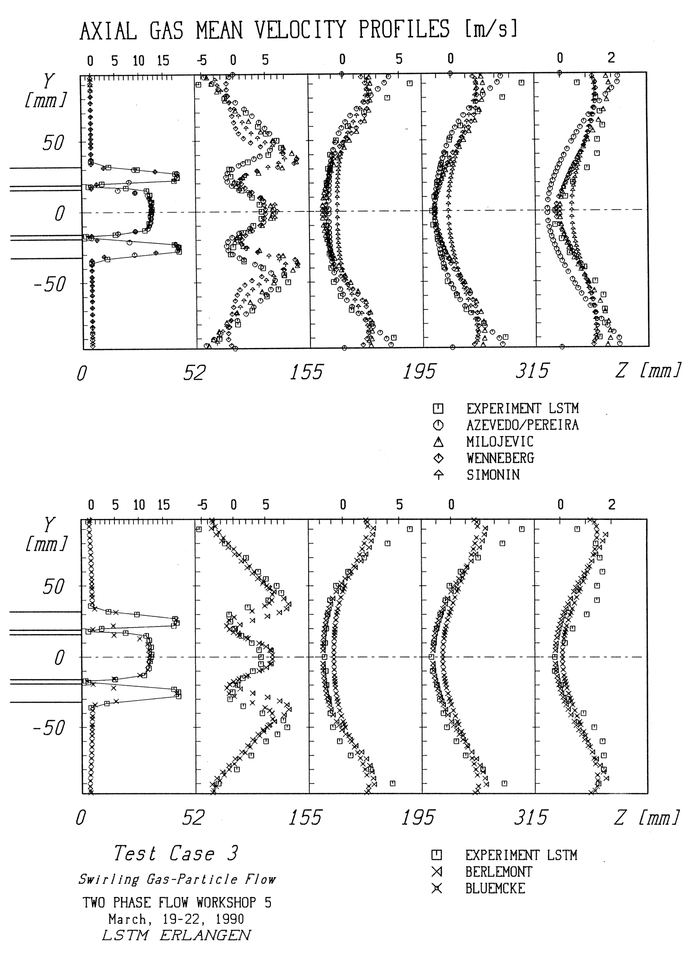Figure 16: Measured and calculated axial gas-phase mean velocity profiles presented at the 5th Workshop on Two-Phase Flow Predictions, Case 1.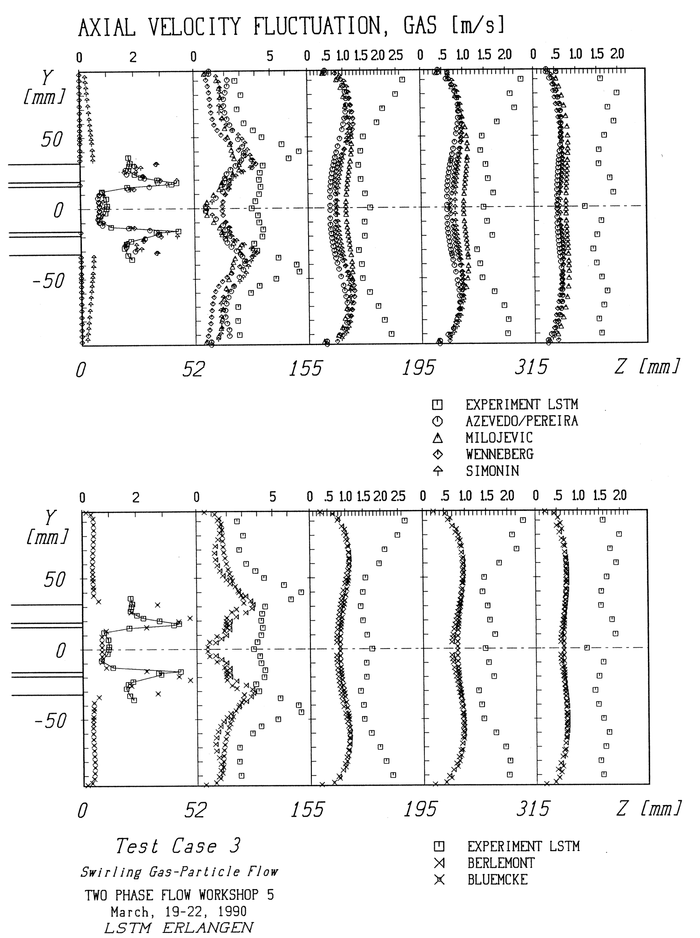Figure 17: Measured and calculated axial gas-phase rms velocity profiles presented at the 5th Workshop on Two-Phase Flow Predictions, Case 1.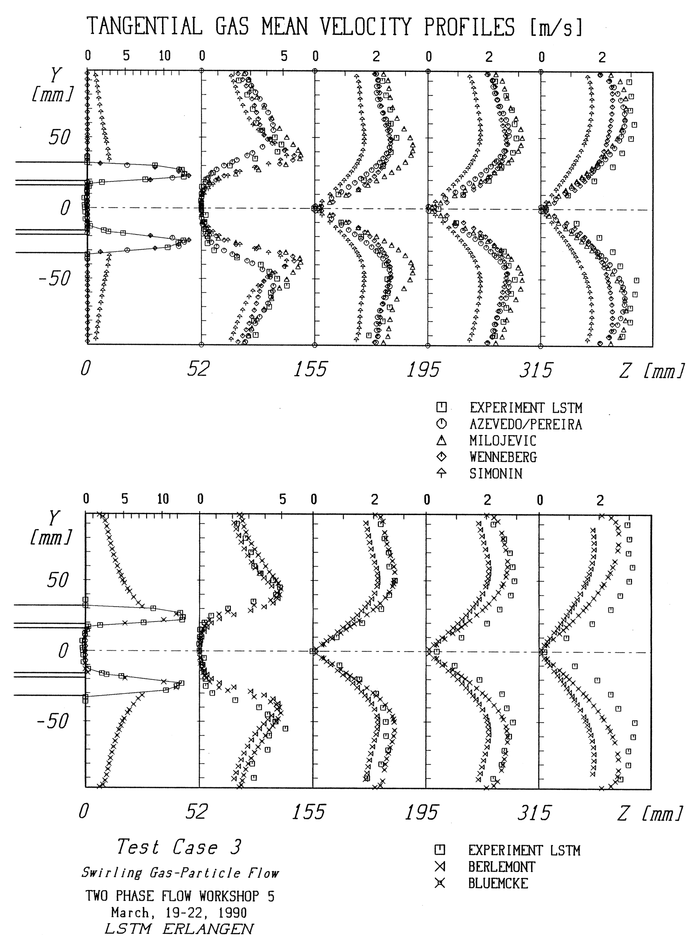Figure 18: Measured and calculated tangential gas-phase mean velocity profiles presented at the 5th Workshop on Two-Phase Flow Predictions, Case 1.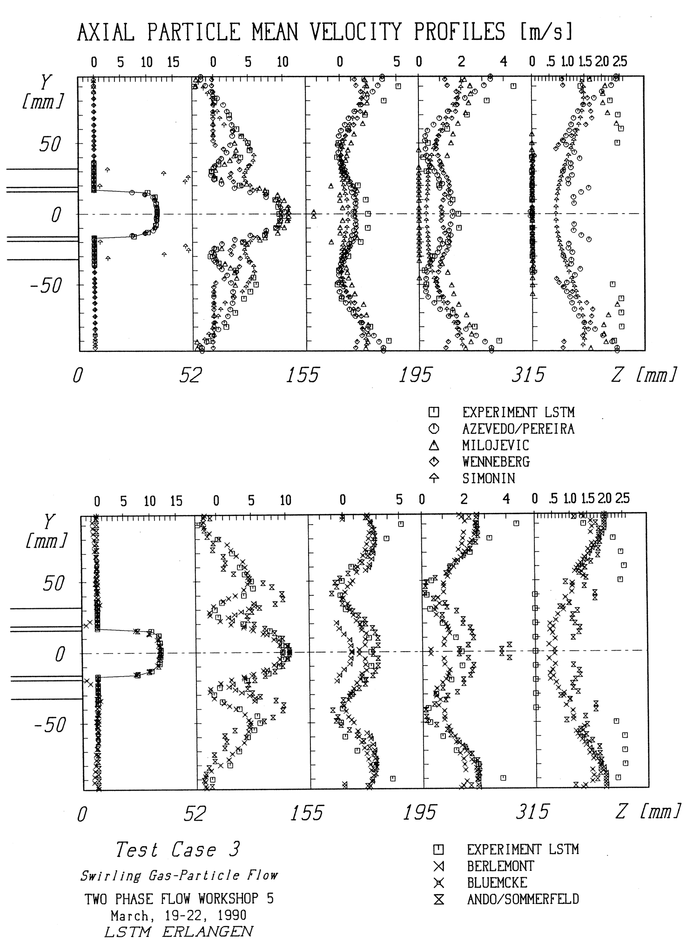Figure 19: Measured and calculated axial particle mean velocity profiles presented at the 5th Workshop on Two-Phase Flow Predictions, Case 1.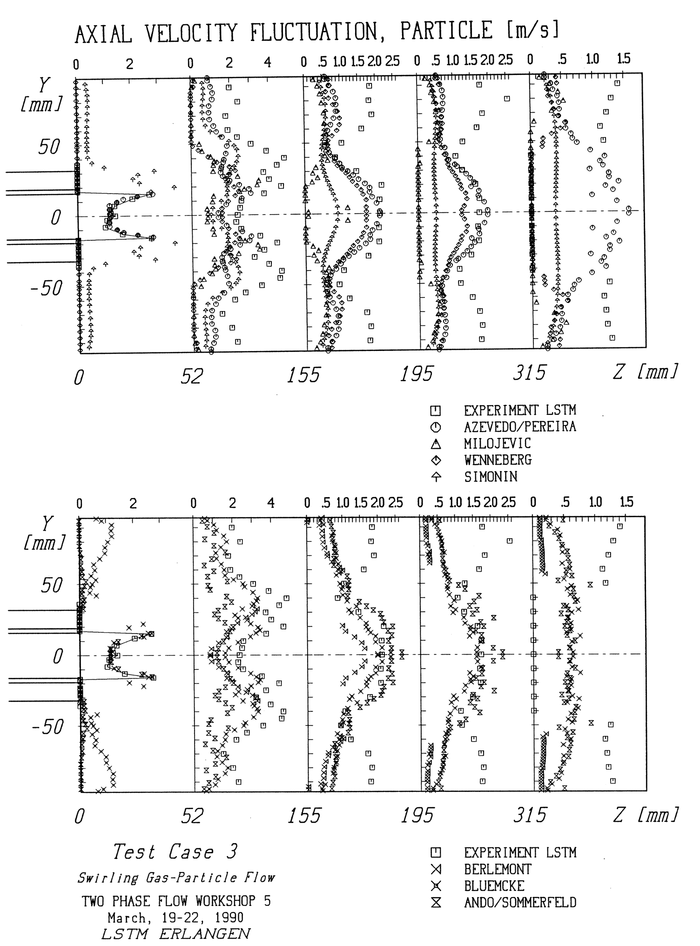Figure 20: Measured and calculated axial particle rms velocity profiles presented at the 5th Workshop on Two-Phase Flow Predictions, Case 1.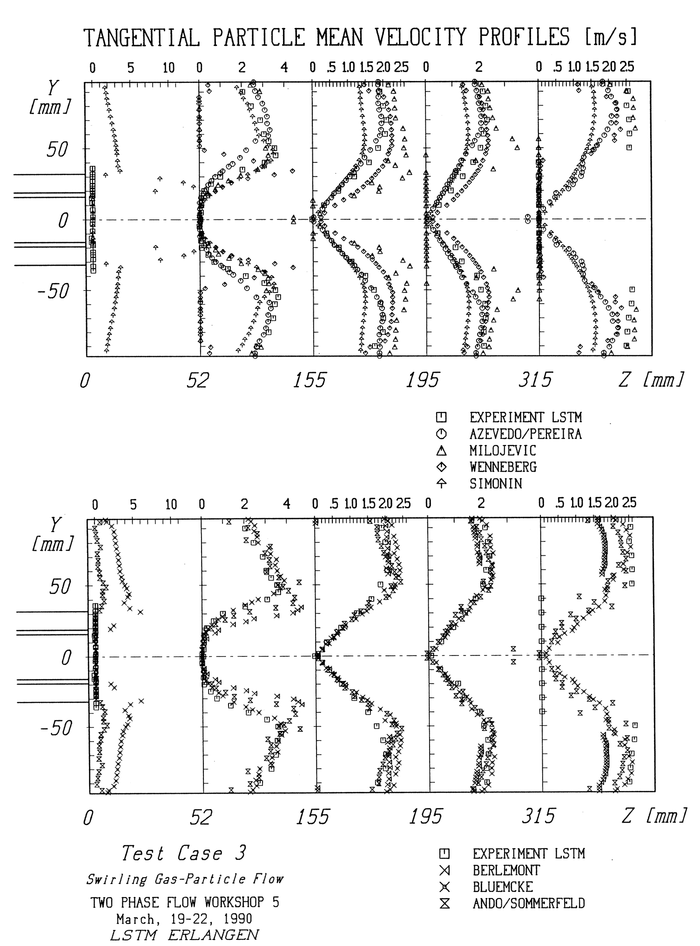Figure 21: Measured and calculated tangential particle-phase mean velocity profiles presented at the 5th Workshop on Two-Phase Flow Predictions, Case 1.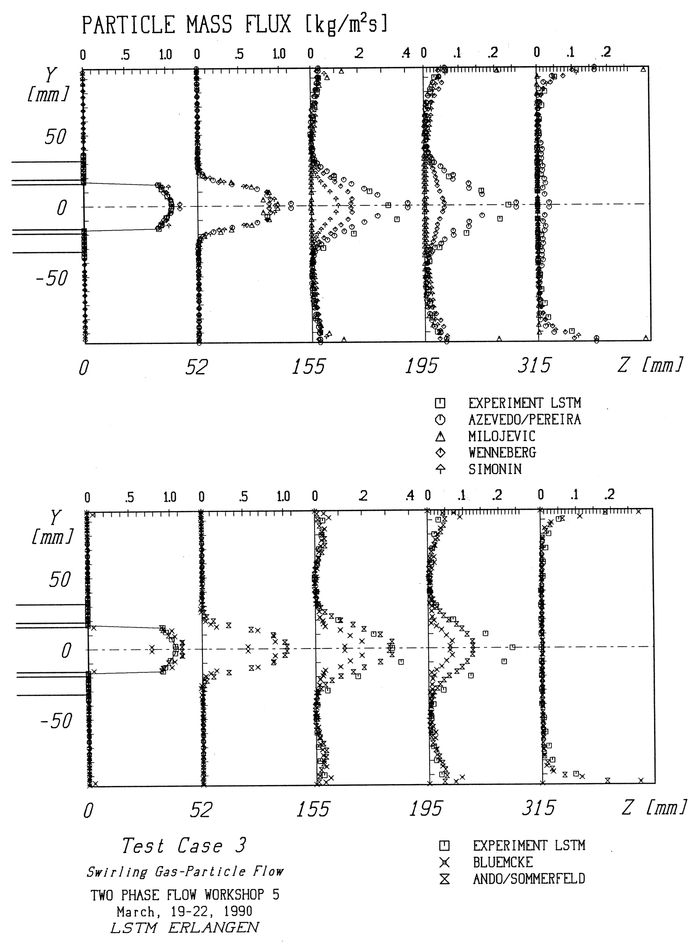Figure 22: Measured and calculated particle mass flux in the stream-wise direction presented at the 5th Workshop on Two-Phase Flow Predictions, Case 1.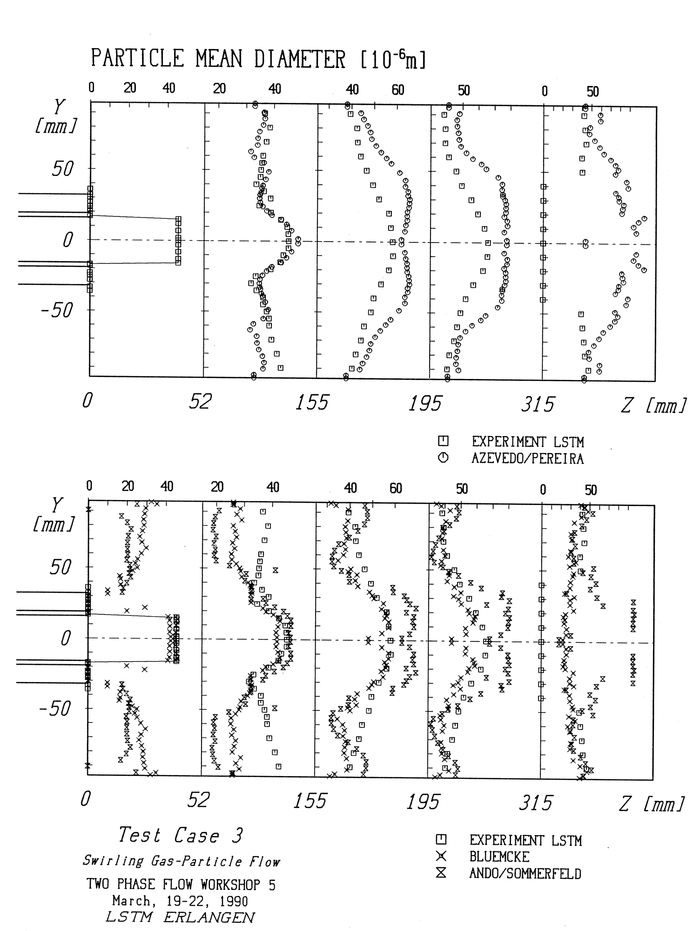Figure 23: Measured and calculated particle mean number diameter presented at the 5th Workshop on Two-Phase Flow Predictions, Case 1.

Contributed by: Martin Sommerfeld — Martin-Luther-Universität Halle-Wittenberg